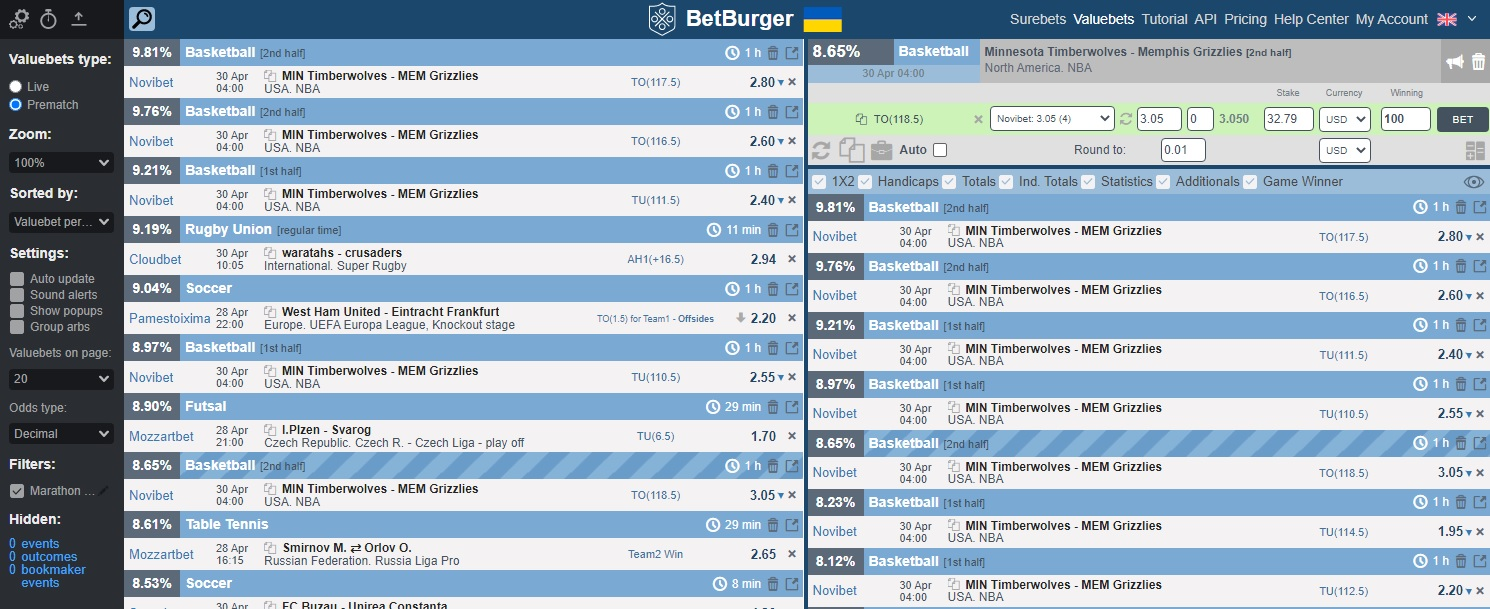Connect with us

# What is the Kelly Criterion in football betting?

Kelly criterion in football betting: ✔ Description of the strategy.

•In the world of betting, there are many different strategies that allow players to increase the chances of winning on bets. The Kelly Criterion financial betting strategy is also quite popular among players. In this article, we will deal with this betting system.

### What is the “Kelly Criterion”

The Kelly Criterion is a financial strategy for sports betting. This system is based on managing the game bank and is a bit like value betting. The essence of strategy – is the correct calculation of the size of each next bet, depending on the results of the previous bet. The player must independently guess the probability of “passing” outcomes, and then to determine the bet size using a special formula. Experts assure that the “Kelly Criterion” saves the bettor’s bank from losing because of no fixed bet.

### How the Kelly strategy works

This system is based on the provision that the player independently determines the probability of the outcome. The bettor must estimate the probability of the selected event, and on the basis of this allocate some part from his bank for a bet. To calculate the size of each bet, 3 conditions are taken into account:

1. Bankroll size.
2. Bookmaker odds.
3. The chances of success of the outcome, chosen by the player (the probability must be higher than set by the bookmaker).

To determine the bet size, you need to use the formula: Sum = В х ((K х V – 1) / (K – 1)) х U

where Sum is the bet amount, B is the bankroll, K is the bookmaker’s odds, V is the probability of the outcome, U is the sum of the bets won.

The sum of the won bets is calculated quite simply: if from the last 100 bets you have won 52, then U = 0.52. Newbies are advised to use the percentage of successful bets with the parameter “0.4”.

Despite the simplicity of the formula, the main problem here is the difficulty of the calculation of the real probability of the outcome. In order to determine it, the player must have great experience and knowledge in sports betting.

However, there are some services, for automatically searching for the outcomes that are more likely to win than think bookmakers. Eg. BetBurger value betting software. This service analyzes the betting lines of more than 200 bookmakers, indicates value bets, and immediately provides their list to the clients.### Calculation example

Let’s consider the Kelly formula on a real example: the football match from 1/4 finals of the 2021–22 UEFA Europa Conference League play-offs between Leicester City and PSV. We are interested in the outcome “Both to score”, which has a probability odd 1.725 or 57.9%. The probability estimated by us is about 70%, so we can see the prospects of bet.

Let’s calculate the size of the bet according to the “Kelly Criterion” for the selected value. To simplify the calculation we set 1000 GBP bankroll and the percent of successful bets in the standard “0.4”.

Sum = 1000 х ((1.725 х 0.7 – 1) / (1.725 – 1)) х 0.4 = 60.17 GBP.

Thanks to the above-mentioned Kelly formula, we found that with a bank of £1000 it would be wise to bet just over £60 on Both To Score.### Conclusion

The Kelly Criterion strategy is mainly used by experienced bettors because newbies often neglect certain important strategy rules. Moreover, you must have real experience in betting to define correctly the real probability of the outcome.

The “Kelly Criterion” is something like a competition between players and bookmakers in the correctness of determining the chance of outcomes passing. The bettor needs always to look for the events underestimated by the bookmakers.

As you understand, the wrong determining of the probability of events can only bring losses. Therefore, if you still can’t analyze events correctly, you can always use the BetBurger service, which will find and calculate the most profitable outcomes in a matter of seconds.